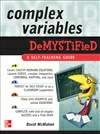# Complex Variables Demystified: A Self-Teaching Guide

Take the complication out of COMPLEX VARIABLES

Ready to learn the fundamentals of complex variables but can't seem to get your brain to function on the right level? No problem! Add Complex Variables Demystified to the equation and you'll exponentially increase your chances of understanding this fascinating subject.

Written in an easy-to-follow format, this book begins by covering complex numbers, functions, limits, and continuity, and the Cauchy-Riemann equations. You'll delve into sequences, Laurent series, complex integration, and residue theory. Then it's on to conformal mapping, transformations, and boundary value problems. Hundreds of examples and worked equations make it easy to understand the material, and end-of-chapter quizzes and a final exam help reinforce learning.

This fast and easy guide offers:

• Numerous figures to illustrate key concepts
• Sample problems with worked solutions
• Coverage of Cauchy-Riemann equations and the Laplace transform
• Chapters on the Schwarz-Christoffel transformation and the gamma and zeta functions
• A time-saving approach to performing better on an exam or at work

Simple enough for a beginner, but challenging enough for an advanced student, Complex Variables Demystified is your integral tool for understanding this essential mathematics topic.

# 下载地址

• 本书版权归原作者所有，本站暂无下载，请购买正版！
•